# Algebra 1 : How to divide monomial quotients

## Example Questions

### Example Question #75 : Monomials

Divide the following monomial quotients: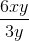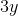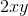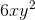Explanation:

To solve this problem, split it into two steps:

1. Divide the coefficients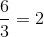2. Divide the variables. We also need to remember the following laws of exponents rule: When dividing variables, subtract the exponents.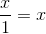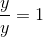Combine these to get the final answer:### Example Question #76 : Monomials

Divide the following monomial quotients: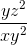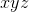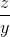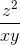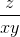Explanation:

To solve this problem, divide the variables. We also need to remember the following laws of exponents rule: When dividing variables, subtract the exponents.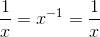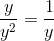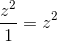Combine these to get the final answer:### Example Question #77 : Monomials

Divide the following monomial quotients: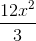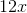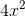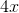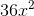Explanation:

To solve this problem, split it into two steps:

1. Divide the coefficients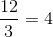2. Divide the variables. We also need to remember the following laws of exponents rule: When dividing variables, subtract the exponents.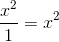Combine these to get the final answer:### Example Question #78 : Monomials

Divide the following monomial quotients: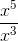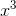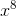Explanation:

To solve this problem, divide the variables. We also need to remember the following laws of exponents rule: When dividing variables, subtract the exponents.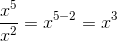Combine these to get the final answer:### Example Question #79 : Monomials

Divide the following monomial quotients: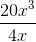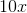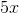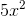Explanation:

To solve this problem, split it into two steps:

1. Divide the coefficients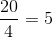2. Divide the variables. We also need to remember the following laws of exponents rule: When dividing variables, subtract the exponents.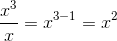Combine these to get the final answer:### Example Question #80 : Monomials

Divide the following monomial quotients: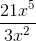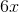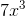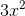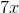Explanation:

To solve this problem, split it into two steps:

1. Divide the coefficients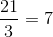2. Divide the variables. We also need to remember the following laws of exponents rule: When dividing variables, subtract the exponents.Combine these to get the final answer:### Example Question #81 : Monomials

Divide the following monomial quotients: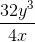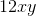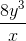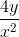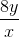Explanation:

To solve this problem, split it into two steps:

1. Divide the coefficients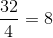2. Divide the variables. We also need to remember the following laws of exponents rule: When dividing variables, subtract the exponents.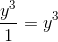Combine these to get the final answer:### Example Question #82 : Monomials

Divide the following monomial quotients: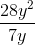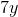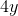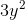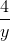Explanation:

To solve this problem, split it into two steps:

1. Divide the coefficients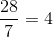2. Divide the variables. We also need to remember the following laws of exponents rule: When dividing variables, subtract the exponents.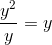Combine these to get the final answer:### Example Question #83 : Monomials

Divide the following monomial quotients: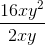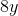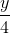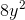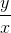Explanation:

To solve this problem, split it into two steps:

1. Divide the coefficients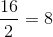2. Divide the variables. We also need to remember the following laws of exponents rule: When dividing variables, subtract the exponents.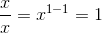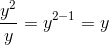Combine these to get the final answer:### Example Question #84 : Monomials

Divide the following monomial quotients: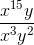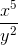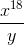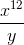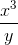Explanation:

To solve this problem, divide the variables. We also need to remember the following laws of exponents rule: When dividing variables, subtract the exponents.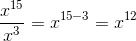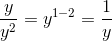Combine these to get the final answer: